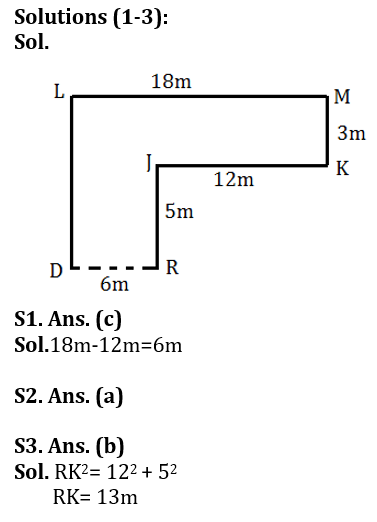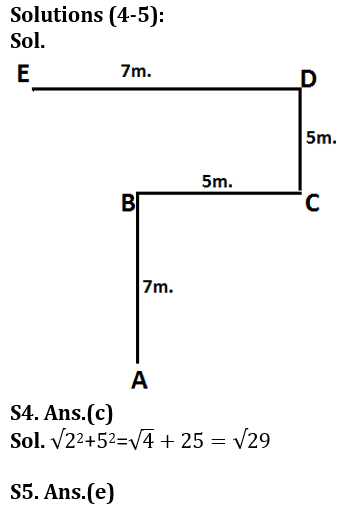Latest Banking jobs   »

# Reasoning Ability Quiz For IDBI AM/ Bank of India PO 2023 -7th March

Point J is 5m north of point R. Point K is 12m east of point J. Point M is 3m north of point K. Point L is 18m west of point M. Point D and R lies in a straight line horizontally. Point D is south of point L.

Q1. What is the distance between point D and R?
(a) 10m
(b) 8m
(c) 6m
(d) 2m
(e) 5m

Q2. In which direction is point L with respect to J?
(a) North-west
(b) South-west
(c) South-east
(d) North-east
(e) None of these

Q3. What is the shortest distance between point K and R?
(a) 10m
(b) 13m
(c) 15m
(d) 2m
(e) 5m

Directions (4-5): Study the information carefully and answer the questions given below.
A person started walking from point A towards north direction after walking of 7m. He reached point B than take a right turn and reached point C after walking 5m. From there he takes a left turn after walking of 5m reach point D and again take a left turn after walking 7m. He reached point E.

Q4. What is the shortest distance between point E to B?
(a) 29m.
(b) 25m.
(c) √29m.
(d) 5√2m.
(e) None of these.

Q5. In which direction is point A with respect to E?
(a) North
(b) South
(c) East
(d) West
(e) None of these.

Directions: (6-10): In these questions, relationship between different elements is shown in the statements. These statements are followed by three/four conclusions. Read the statements and then decide which of the following conclusions follow from the given statements.

Q6. Statements: D≤M>O, K≥T, D>T
Conclusions: I. T<M
II. K=D
III. O<K
(a) Only I follows
(b) Only II follows
(c) Only III follows
(d) Only I and II follow
(e) None of these

Q7. Statements: S<T≤U, T>P, I=T≤D
Conclusions: I. I>P
II. U≥D
III. S≤D
(a) Only III follows
(b) Only I and II follows
(c) Only I follows
(d) Only II and III follow
(e) None of these

Q8. Statements: F<I≤R, P≥L=I>C=E
Conclusions: I. R>E
II. P>F
III. P≥C
(a) Only I and II follow
(b) Only II and III follow
(c) Only I and III follow
(d) All follow
(e) None of these

Q9. Statements: S≥T, D>E, N≥T, U>E≥N
Conclusions: I. N≤U
II. S>E
III. T≤D
(a) Only I and II follow
(b) Only II and III follow
(c) Only I follows
(d) Only III follows
(e) None of these

Q10. Statements: K=A<T, P<U, U≥G≥T
Conclusions: I. A>P
II. G≥K
III. U≥T
(a) Only I and II follows
(b) Only II and III follows
(c) Only I follows
(d) Only III follows
(e) All follow

Directions (11-15): In these questions, a relationship between different elements is shown in the statements. The statements are followed by two conclusions. Give answer

Q11. Statement: G<D≤E; G≥F>B<A
Conclusion: I. G≥A II. B<E
(a) if only conclusion II is true.
(b) if only conclusion I is true.
(c) if neither conclusion I nor II is true.
(d) if either conclusion I or II is true.
(e) if both conclusions I and II are true.

Q12. Statement: K=A, A>T≥J; A≤S=H; I>T
Conclusion: I. H>J II. I>S
(a) if both conclusion I and II are true.
(b) if only conclusion I is true.
(c) if neither conclusion I nor II is true.
(d) if either conclusion I or II is true.
(e) if only conclusion II is true.

Q13. Statement: P>J≥K>L, L<M≥N; K≥O=T
Conclusion: I. J>O II. J=T
(a) if only conclusion II is true.
(b) if either conclusion I or II is true.
(c) if neither conclusion I nor II is true.
(d) if only conclusion I is true.
(e) if both conclusions I and II are true.

Q14. Statement: O < A> T=N; A>S>R; A<M>P
Conclusion: I. R<T II. N≤R
(a) if only conclusion II is true.
(b) if only conclusion I is true.
(c) if neither conclusion I nor II is true.
(d) if either conclusion I or II is true.
(e) if both conclusions I and II are true.

Q15. Statement: A<B≤C; E > F<M≤C; C>Q<R
Conclusion: I. Q≤F II. F>Q
(a) if only conclusion II is true.
(b) if either conclusion I or II is true.
(c) if neither conclusion I nor II is true.
(d) if only conclusion I is true.
(e) if both conclusions I and II are true.

SolutionsSolutions (6-10):
S6. Ans. (a)
S7. Ans. (c)
S8. Ans. (a)
S9. Ans. (e)
S10. Ans. (d)

Solutions (11-15):
S11. Ans. (a)
Sol. I. G≥A(False) II. B<E(True)

S12. Ans. (b)
Sol. I. H>J(True) II. I>S (False)

S13. Ans. (b)
Sol. I. J>O(False) II. J=T (False)

S14. Ans. (d)
Sol. I. R<T(False) II. N≤R(False)

S15. Ans. (c)
Sol. I. Q≤F (False) II. F>Q(False)## FAQs

### What is the selection process of the IDBI AM?

The selection process of IDBI AM is Online exam and Interview.

#### Congratulations!Union Budget 2023-24: Free PDF